Important Coordinate Geometry Questions

Chapter 7 Class 10 Coordinate Geometry
Serial order wise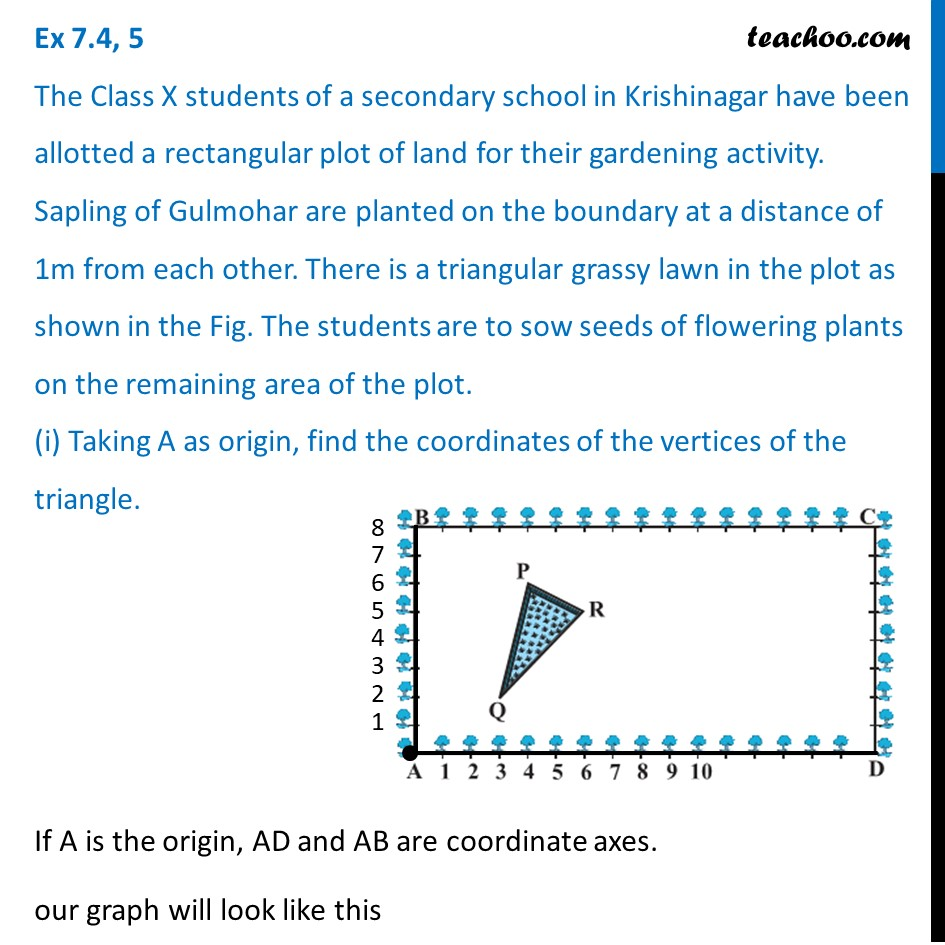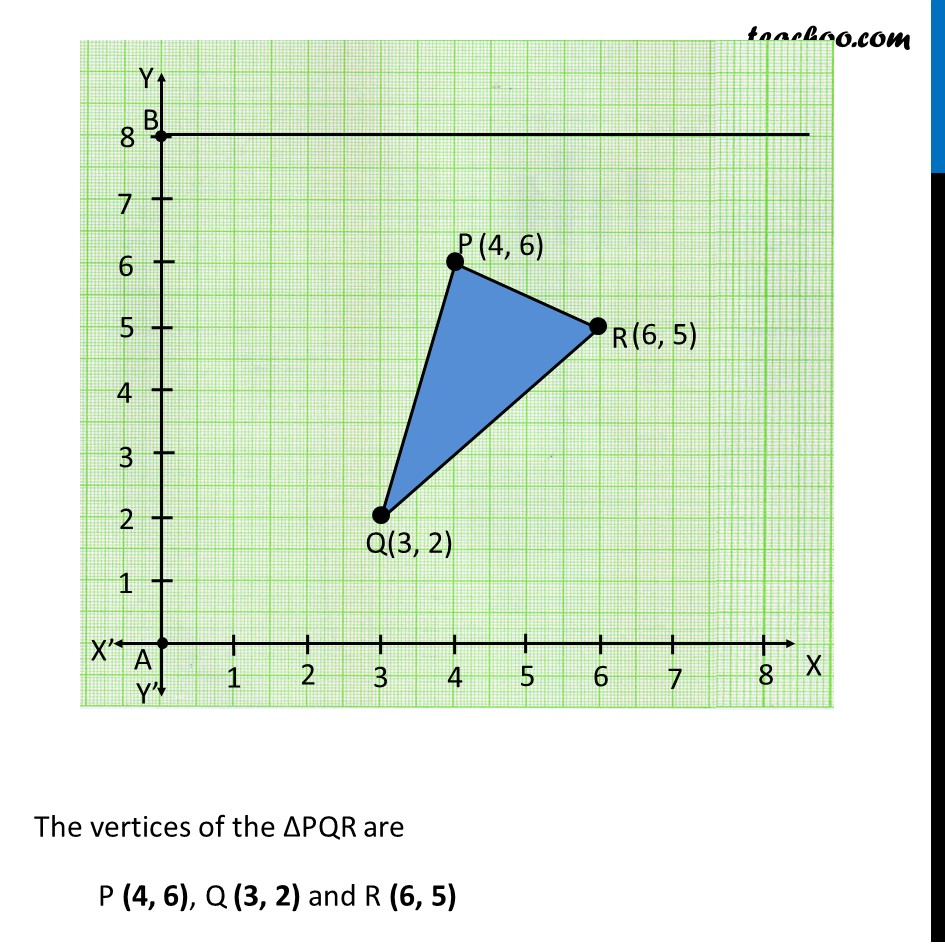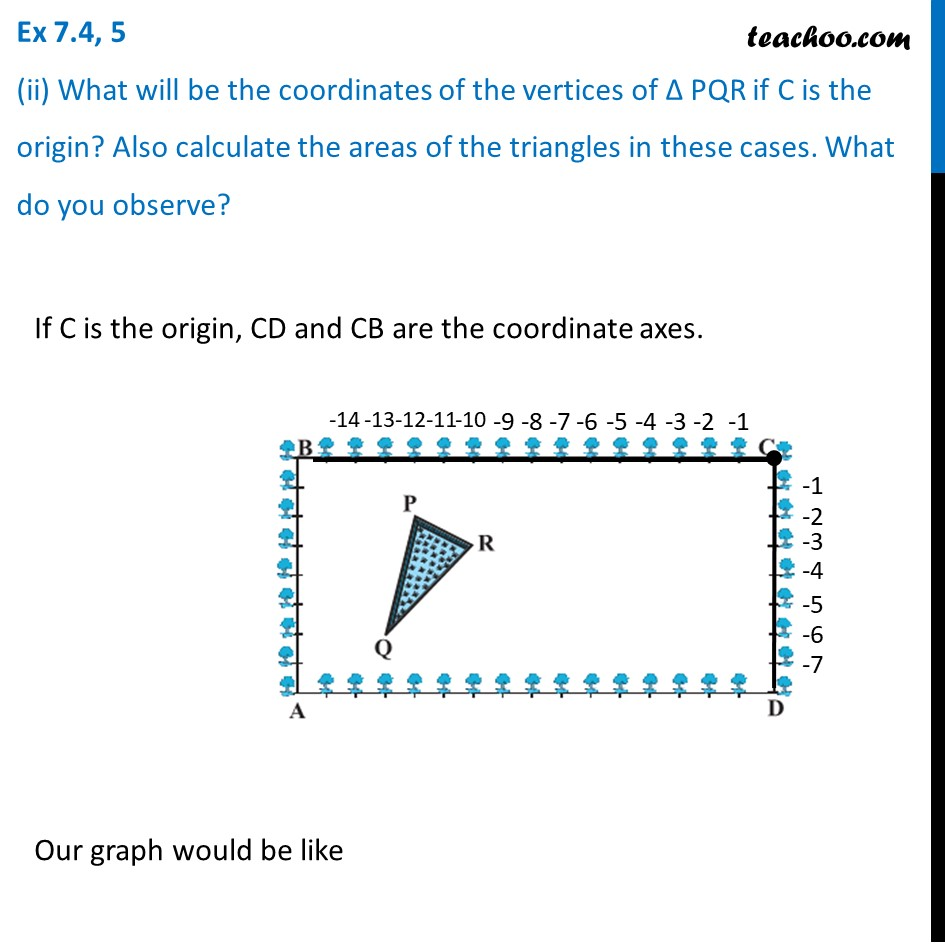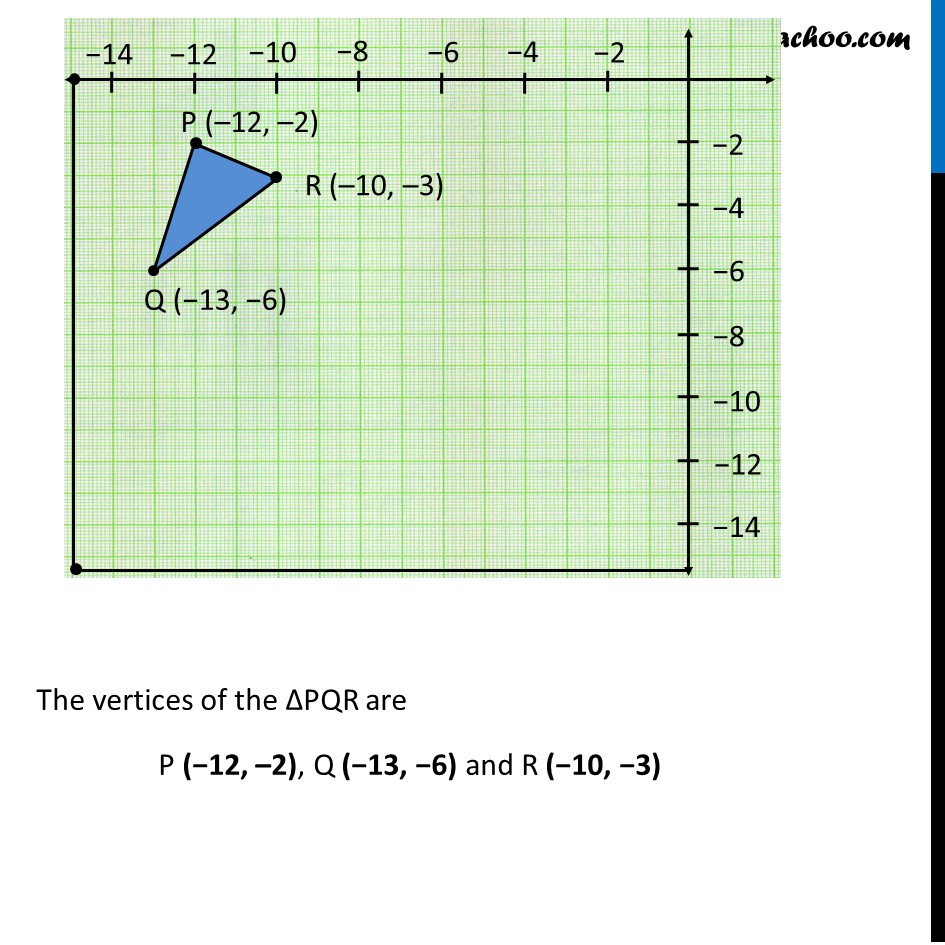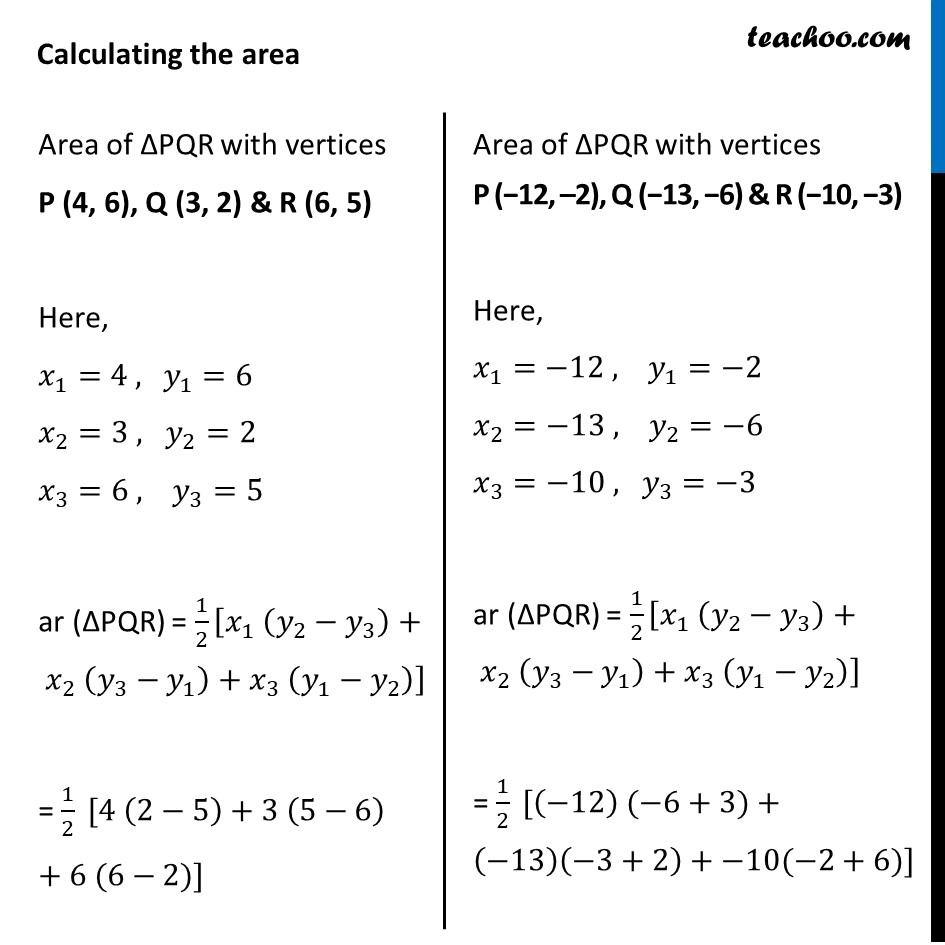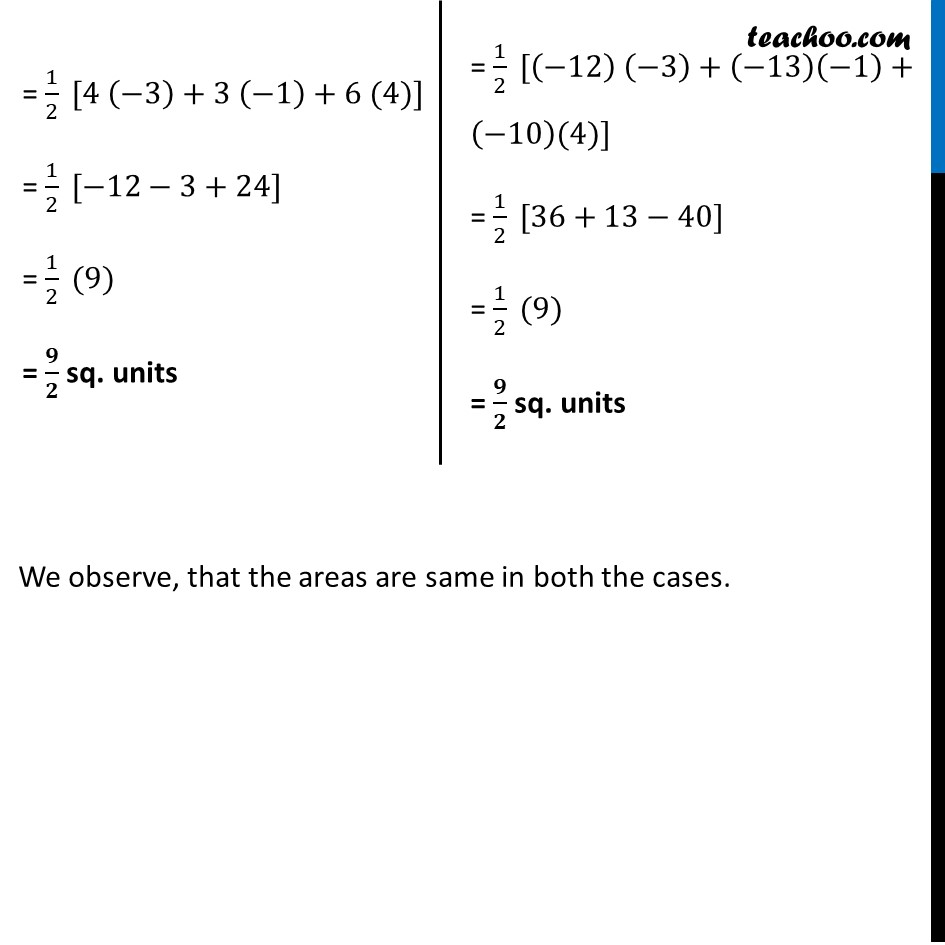Learn in your speed, with individual attention - Teachoo Maths 1-on-1 Class

### Transcript

Question 5 The Class X students of a secondary school in Krishinagar have been allotted a rectangular plot of land for their gardening activity. Sapling of Gulmohar are planted on the boundary at a distance of 1m from each other. There is a triangular grassy lawn in the plot as shown in the Fig. The students are to sow seeds of flowering plants on the remaining area of the plot. (i) Taking A as origin, find the coordinates of the vertices of the triangle. If A is the origin, AD and AB are coordinate axes. our graph will look like this The vertices of the ∆PQR are P (4, 6), Q (3, 2) and R (6, 5) Question 5 (ii) What will be the coordinates of the vertices of Δ PQR if C is the origin? Also calculate the areas of the triangles in these cases. What do you observe? If C is the origin, CD and CB are the coordinate axes. Our graph would be like The vertices of the ∆PQR are P (−12, –2), Q (−13, −6) and R (−10, −3) Calculating the area Area of ∆PQR with vertices P (4, 6), Q (3, 2) & R (6, 5) Here, 𝑥_1=4 , 〖 𝑦〗_1=6 𝑥_2=3 , 𝑦_2=2 𝑥_3=6 , 𝑦_3=5 ar (∆PQR) = 1/2 [𝑥_1 (𝑦_2−𝑦_3 )+ 𝑥_2 (𝑦_3−𝑦_1 )+𝑥_3 (𝑦_1−𝑦_2 )] = 1/2 [4 (2−5)+3 (5−6) + 6 (6−2)] Area of ∆PQR with vertices P (−12, –2), Q (−13, −6) & R (−10, −3) Here, 𝑥_1=−12 , 𝑦_1=−2 𝑥_2=−13 , 𝑦_2=−6 𝑥_3=−10 , 𝑦_3=−3 ar (∆PQR) = 1/2 [𝑥_1 (𝑦_2−𝑦_3 )+ 𝑥_2 (𝑦_3−𝑦_1 )+𝑥_3 (𝑦_1−𝑦_2 )] = 1/2 [(−12) (−6+3)+(−13)(−3+2)+−10(−2+6)] = 1/2 [4 (−3)+3 (−1)+6 (4)] = 1/2 [−12−3+24] = 1/2 (9) = 𝟗/𝟐 sq. units = 1/2 [(−12) (−3)+(−13)(−1)+(−10)(4)] = 1/2 [36+13−40] = 1/2 (9) = 𝟗/𝟐 sq. units = 1/2 [(−12) (−3)+(−13)(−1)+(−10)(4)] = 1/2 [36+13−40] = 1/2 (9) = 𝟗/𝟐 sq. units We observe, that the areas are same in both the cases.